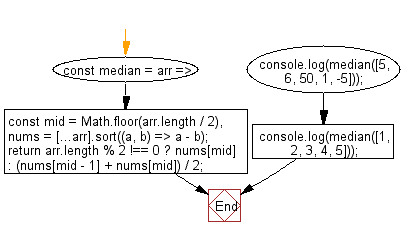# JavaScript: Get the median of an array of numbers

## JavaScript fundamental (ES6 Syntax): Exercise-88 with Solution

Write a JavaScript program to get the median of an array of numbers.

Note: Find the middle of the array, use Array.sort() to sort the values. Return the number at the midpoint if length is odd, otherwise the average of the two middle numbers.

• Find the middle of the array, use Array.prototype.sort() to sort the values.
• Return the number at the midpoint if Array.prototype.length is odd, otherwise the average of the two middle numbers.

Sample Solution:

JavaScript Code:

``````//#Source https://bit.ly/2neWfJ2
const median = arr => {
const mid = Math.floor(arr.length / 2),
nums = [...arr].sort((a, b) => a - b);
return arr.length % 2 !== 0 ? nums[mid] : (nums[mid - 1] + nums[mid]) / 2;
};
console.log(median([5, 6, 50, 1, -5]));
console.log(median([1, 2, 3, 4, 5]));
```
```

Sample Output:

```5
3
```

Pictorial Presentation:Flowchart:Live Demo:

See the Pen javascript-basic-exercise-88-1 by w3resource (@w3resource) on CodePen.

Improve this sample solution and post your code through Disqus

What is the difficulty level of this exercise?

Test your Programming skills with w3resource's quiz.

﻿